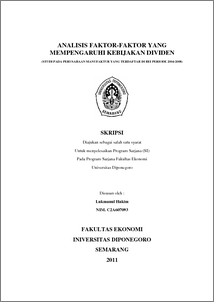# ANALISIS FAKTOR-FAKTOR YANG MEMPENGARUHI KEBIJAKAN DIVIDEN (STUDI PADA PERUSAHAAN MANUFAKTUR YANG TERDAFTAR DI BEI PERIODE 2004-2008)

HAKIM, Lukmanul and TRI WIDYARTI, Endang (2011) ANALISIS FAKTOR-FAKTOR YANG MEMPENGARUHI KEBIJAKAN DIVIDEN (STUDI PADA PERUSAHAAN MANUFAKTUR YANG TERDAFTAR DI BEI PERIODE 2004-2008). Undergraduate thesis, Universitas Diponegoro.Preview
PDF
325Kb

## Abstract

This research was conducted to examine the effect of variable cash ratio, growth, size, Retun on Assets (ROA), and Debt to Total Asset (DTA) on Dividend Payout Ratio on companies listed on the Jakarta Stock Exchange 2004-2008 period. The sampling technique used is pusposive sanpling and the number of samples used for as many as 9 companies. The variables used in this study, namely the dividend payout ratio (DPR) as the dependent variable and the return on assets (ROA), cash ratio (CR), leverage ratio (LR), growth, size as independent variables. Data obtained from the publication of Indonesian Capital Market Directory (ICMD 2004, 2005, 2006, 2007, and 2008). The analysis technique used is multiple regression analysis and hypothesis testing using the t statistic for testing the partial regression coefficient and F-statistic to test the effect together with the 5% level of confidence. The results showed that the cash ratio and size have a positive and not significant to the DPR. This research also found that growth was not significantly negative effect on DPR. Other variables in this study is a Debt to Total Asset (DTA) and Return on Assets (ROA) has positive and significant impact on the DPR. Of the 5 hypothesis that there is only one hypothesis is rejected is the third hypothesis, DTA negatively related to the DPR, because after the regression test results contradict the hypothesis that DTA positively related to the DPR. Based on a statistical analysis of significance of F values obtained 0,000 (less than 0.05), so it can be concluded that the variables ROA, CR, DTA, Growth, and Size have a significant influence on DPR. From Adjusted R2 value of 0.356 indicates that there are independent variables in the model can explain the variation the DPR by 35.6%, while 64.4% variation explained by the House of Representatives of other variables outside the model.

Item Type: Thesis (Undergraduate) Cash ratio, growth, size, Return On Asset, debt to total asset, dividend payout ratio Cash ratio, growth, size, Return On Asset, debt to total asset, dividend payout ratio H Social Sciences > HD Industries. Land use. Labor > HD28 Management. Industrial Management Faculty of Economics and Business > Department of Management 26842 INVALID USER 19 Apr 2011 09:43 19 Apr 2011 09:43

Repository Staff Only: item control page